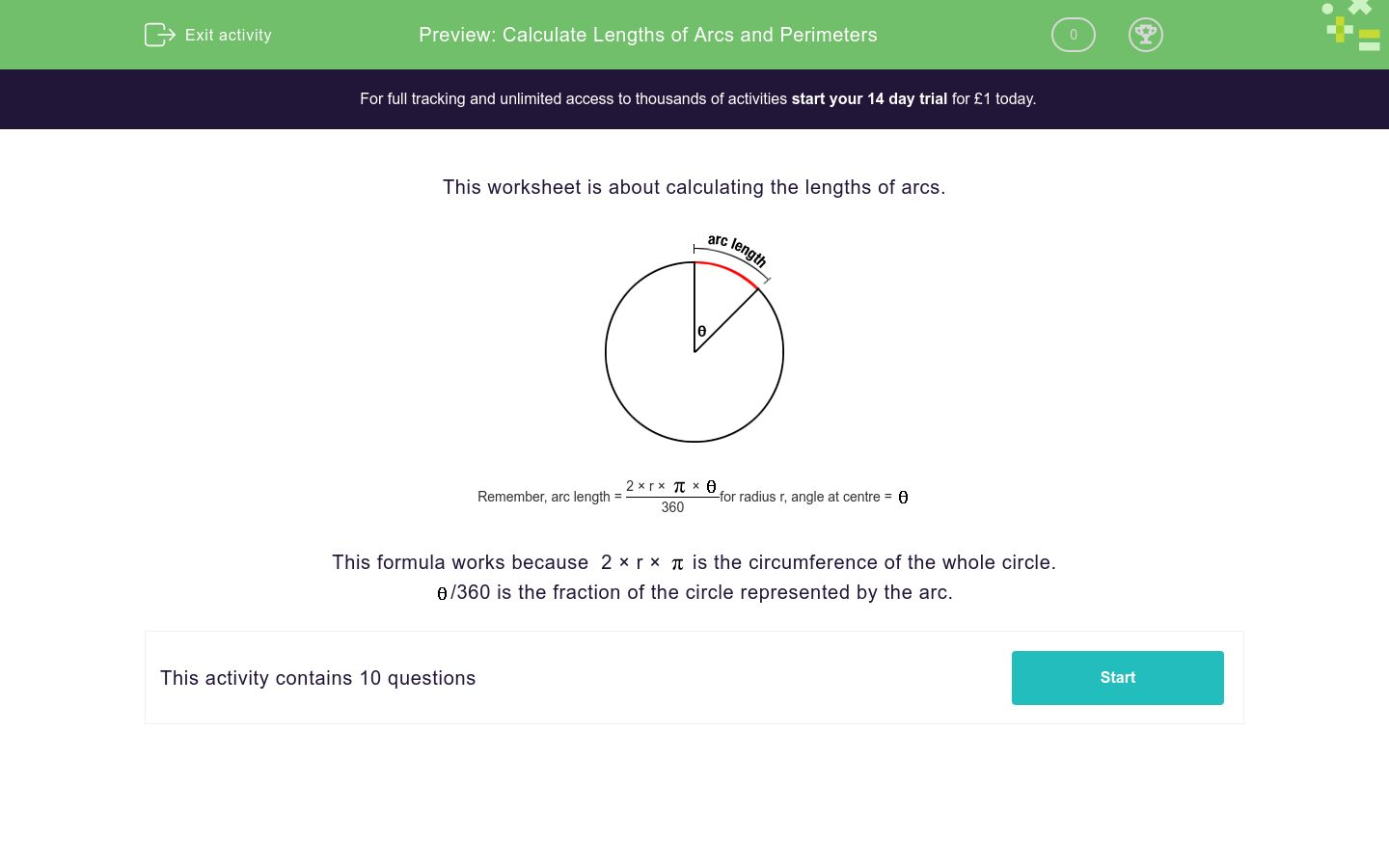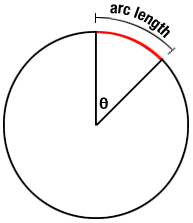# Calculate Lengths of Arcs and Perimeters

In this worksheet, students calculate lengths of arcs and perimeters of circular shapes.Key stage:  KS 4

Curriculum topic:  Geometry and Measures

Curriculum subtopic:  Calculate Arc Lengths, Angles and Areas of Sectors of Circles

Difficulty level:### QUESTION 1 of 10

This worksheet is about calculating the lengths of arcs.Remember, arc length = 2 × r ××for radius r, angle at centre =360

This formula works because  2 × r ×is the circumference of the whole circle./360 is the fraction of the circle represented by the arc.

Calculate the arc length of the given shape to 3 sig. figs.

 Remember, arc length = 2 × r ××for radius r, angle at centre =360R = 24 cm

Θ = 11.5 °

Arc length = ____ cm

Calculate the arc length of the given shape to 3 sig. figs.

 Remember, arc length = 2 × r ××for radius r, angle at centre =360R = 22.5 cm

Θ = 9°

Arc length = ____ cm

Calculate the perimeter of the given shape to 3 sig. figs.

 Remember, arc length = 2 × r ××for radius r, angle at centre =360R = 6 cm

Θ = 8.5°

Perimeter = ____ cm

Calculate the perimeter of the given shape to 3 sig. figs.

 Remember, arc length = 2 × r ××for radius r, angle at centre =360a = 10 cm

b = 5 cm

r = 8.9 cm

φ = 40°

Perimeter = ____ cm

Calculate the perimeter of the given shape to 3 sig. figs.

 Remember, arc length = 2 × r ××for radius r, angle at centre =360a = 13 cm

b = 3 cm

r = 3.4 cm

φ = 40°

Perimeter = ____ cm

Calculate the perimeter of the given shape to 3 sig. figs.

 Remember, arc length = 2 × r ××for radius r, angle at centre =360R = 5 cm

Θ = 8 °

Perimeter = ____ cm

Calculate the perimeter of the given shape to 3 sig. figs.R = 9.5 cm

Θ = 10 °

Perimeter = ____ cm

Calculate the perimeter of the given shape to 3 sig. figs.a = 15 cm

b = 6 cm

r = 9.9 cm

φ = 55°

Perimeter = ____ cm

Calculate the perimeter of the given shape to 3 sig. figs.R = 11.5 cm

Θ = 10 °

Perimeter = ____ cm

Calculate the perimeter of the given shape to 3 sig. figs.R = 20 cm

Θ = 7 °

Perimeter = ____ cm

• Question 1

Calculate the arc length of the given shape to 3 sig. figs.

 Remember, arc length = 2 × r ××for radius r, angle at centre =360R = 24 cm

Θ = 11.5 °

Arc length = ____ cm

4.82
EDDIE SAYS
 2 × 24 ×× 11.5 360
• Question 2

Calculate the arc length of the given shape to 3 sig. figs.

 Remember, arc length = 2 × r ××for radius r, angle at centre =360R = 22.5 cm

Θ = 9°

Arc length = ____ cm

3.53
EDDIE SAYS
 2 × 22.5 ×× 9 360
• Question 3

Calculate the perimeter of the given shape to 3 sig. figs.

 Remember, arc length = 2 × r ××for radius r, angle at centre =360R = 6 cm

Θ = 8.5°

Perimeter = ____ cm

12.9
EDDIE SAYS
Remember to include the two radii.
 2 × 6 ×× 8.5 + 6 + 6 360
• Question 4

Calculate the perimeter of the given shape to 3 sig. figs.

 Remember, arc length = 2 × r ××for radius r, angle at centre =360a = 10 cm

b = 5 cm

r = 8.9 cm

φ = 40°

Perimeter = ____ cm

31.2
EDDIE SAYS
Remember to include the three sides.
 2 × 8.9 ×× 40 + 10 + 10 + 5 360
• Question 5

Calculate the perimeter of the given shape to 3 sig. figs.

 Remember, arc length = 2 × r ××for radius r, angle at centre =360a = 13 cm

b = 3 cm

r = 3.4 cm

φ = 40°

Perimeter = ____ cm

31.4
EDDIE SAYS
Remember to include the three sides.
 2 × 3.4 ×× 40 + 13 + 13 + 3 360
• Question 6

Calculate the perimeter of the given shape to 3 sig. figs.

 Remember, arc length = 2 × r ××for radius r, angle at centre =360R = 5 cm

Θ = 8 °

Perimeter = ____ cm

10.7
EDDIE SAYS

Remember to include the two radii.

 2 × 5 ×× 8 + 5 + 5 360
• Question 7

Calculate the perimeter of the given shape to 3 sig. figs.R = 9.5 cm

Θ = 10 °

Perimeter = ____ cm

20.7
EDDIE SAYS
Remember to include the two radii.
 2 × 9.5 ×× 10 + 9.5 + 9.5 360
• Question 8

Calculate the perimeter of the given shape to 3 sig. figs.a = 15 cm

b = 6 cm

r = 9.9 cm

φ = 55°

Perimeter = ____ cm

45.5
EDDIE SAYS

Remember to include the three sides.

 2 × 9.9 ×× 55 + 15 + 15 + 6 360
• Question 9

Calculate the perimeter of the given shape to 3 sig. figs.R = 11.5 cm

Θ = 10 °

Perimeter = ____ cm

25.0
EDDIE SAYS

Remember to include the two radii.

 2 × 11.5 ×× 10 + 11.5 + 11.5 360
• Question 10

Calculate the perimeter of the given shape to 3 sig. figs.R = 20 cm

Θ = 7 °

Perimeter = ____ cm

42.4
EDDIE SAYS
Remember to include the two radii.
 2 × 20 ×× 7 + 20 + 20 360
---- OR ----

Sign up for a £1 trial so you can track and measure your child's progress on this activity.

### What is EdPlace?

We're your National Curriculum aligned online education content provider helping each child succeed in English, maths and science from year 1 to GCSE. With an EdPlace account you’ll be able to track and measure progress, helping each child achieve their best. We build confidence and attainment by personalising each child’s learning at a level that suits them.